## Solver Exception Events

### Tolerance-Exceeding Events

This example simulates two identical nonlinear spring-damping systems. The two systems have different spring stiffness.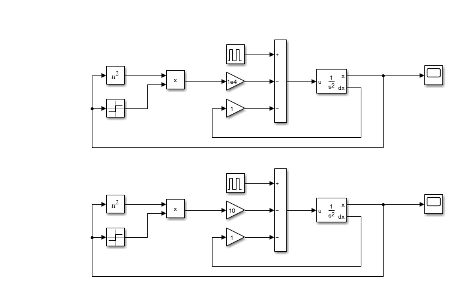When you run the Solver Profiler on this model, you can see the tolerance-exceeding events in the Solver Exception tab.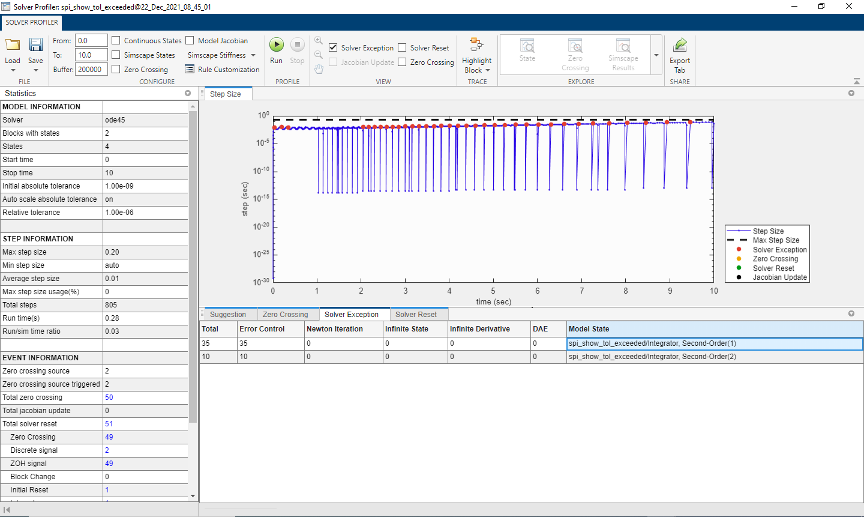The result indicates that the stiffer spring causes the solver tolerance to exceed the limit. Typically, model states that change the fastest tend to be closest to the solver tolerance limit.

The solver attempts to take the largest possible steps while optimally trading off between speed and accuracy. Occasionally, this tradeoff causes the solver to take steps that exceed the tolerance limit and forces it to reduce the step size. Exceeding the tolerance limit is not a poor modeling practice in itself. This profiler statistic is not meant to help you reduce tolerance exceeding events to zero.

This statistic can help you identify parts of your model that are close to exceeding the tolerance limit. You can identify model components that change the fastest or are the stiffest. You can decide to retain this model dynamic in the simulation or simplify it to speed up simulation.

The tolerance-exceeding statistic can also help you identify modeling errors. If you do not expect the highlighted states to change as fast, you can examine your model for errors. In this example, the modeling error could be that the stiffness of the stiffer spring is specified in `N/m` instead of `N/mm`. This error makes the spring 1000 times stiffer than expected.

### Newton Iteration Failures

Newton iteration failures are specific to implicit solvers like `ode15s` and `ode23t`, and they result from Newton iterations not converging after a few trials. Similar to tolerance-exceeding events, these failures tend to occur when a system changes quickly.

This example simulates how the radius of a ball of flame changes when you strike a match. The ball of flame grows rapidly until it reaches a critical size, when the amount of oxygen consumed balances the growth in ball surface.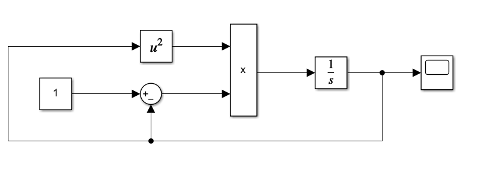When you run the Solver Profiler on this model, you can see the Newton iteration failures in the Solver Exception tab.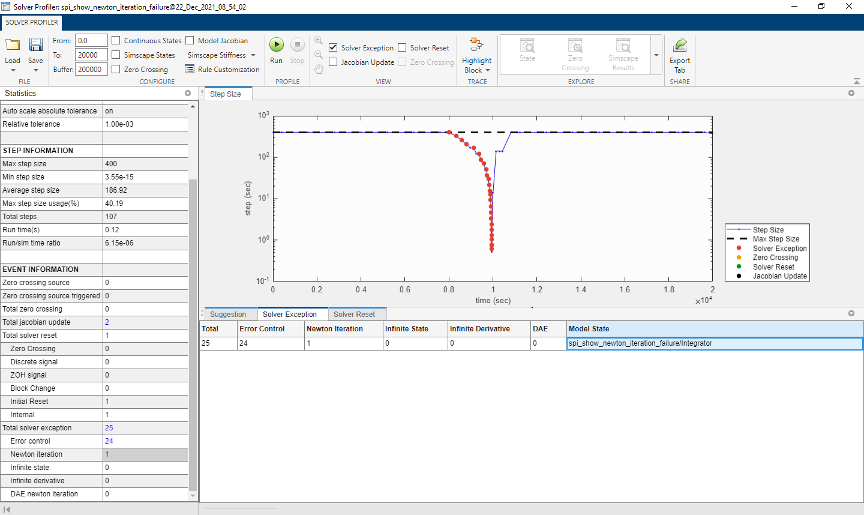The result indicates that when the combustion begins, the solver tolerance is exceeded multiple times. When equilibrium is attained, the system appears different, and a Newton iteration failure occurs. The Jacobian of the system is recomputed, and the solver continues to move forward.

Newton failures are not indicative of poor modeling practices. This profiler statistic is not meant to help you reduce these failures to zero. In this example, you can reduce the solver tolerance to prevent this failure. But the solver then takes small steps unnecessarily, which is counterproductive. Therefore, in this example, this failure is acceptable.

This type of failure becomes problematic when it occurs in large numbers over a short period, especially in Simscape™ models. Dense failures indicate that your model is not robust numerically. One way to improve numerical robustness is to tighten the solver tolerance. Another way is to modify the model to avoid rapid changes.

### Infinite State and Infinite Derivative Exceptions

An infinite state exception occurs when the magnitude of a state approaches infinity. Similarly, when the magnitude of the derivative of a state approaches infinity, an infinite derivative exception occurs. The solver reduces the step size and makes another attempt at integration.

This example shows a set of two Integrator Limited blocks that have initial conditions slightly less than `realmax`. As they integrate the Constant input of `1e305`, the solver quickly reaches the infinite state exception.When you run the Solver Profiler for this model, you can see that the InfiniteState5 and InfiniteState7 blocks have `188` and `152` infinite state exceptions in the Solver Exceptions tab.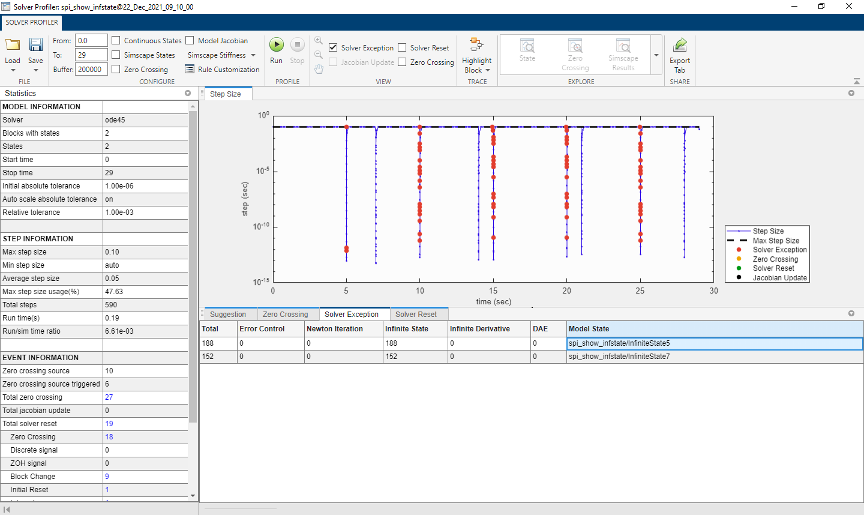### Differential Algebraic Equation Failures

Most Simscape models use differential algebraic equations (DAEs), in contrast to Simulink® models, which use ordinary differential equations.

The use of DAEs adds complexity to Simscape models. Solvers like `ode15s` and `ode23t` can handle many types of DAEs. However, when the algebraic constraints between Simscape components are complex and changing fast, the Newton iteration process fails to resolve to those constraints.

This example simulates a pressure source that can be directed toward one of two hydraulic chambers.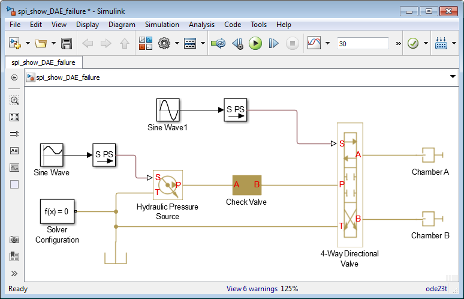When you simulate this model, Simulink displays several warnings.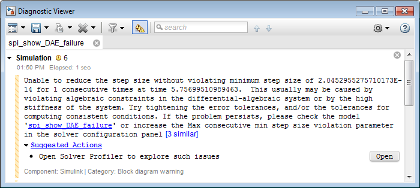Typically, small models can handle such warnings and simulate to completion. However, this warning indicates that the model is not robust numerically. Minor changes to the model, or integration into a larger model, can result in errors.

When you run the Solver Profiler on this model, you can see the DAE failures in the Solver Exception tab.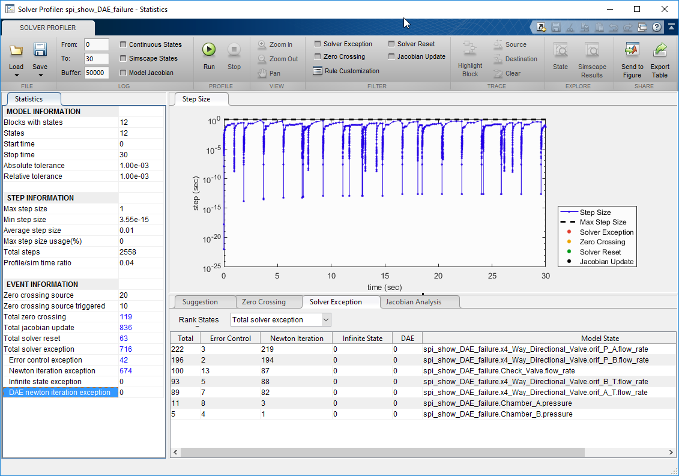In this case, the exception results from large solver tolerance. Tightening the solver tolerance forces the solver to take smaller steps and better capture the changes in algebraic constraints.

Alternatively, this exception can be avoided by removing the algebraic constraint. In this example, the Check Valve and the 4-Way Directional Valve are directly connected. When their pressure–flow relationship changes rapidly, the solver is unable to capture the changes. Inserting a Hydraulic Chamber between those two components makes them compliant. To learn more about dry nodes, see Simscape documentation.